Shortcuts

# Parameter Scheduler¶

During neural network training, optimization hyperparameters (e.g. learning rate) are usually adjusted along with the training process. One of the simplest and most common learning rate adjustment strategies is multi-step learning rate decay, which reduces the learning rate to a fraction at regular intervals. PyTorch provides LRScheduler to implement various learning rate adjustment strategies. In MMEngine, we have extended it and implemented a more general ParamScheduler. It can adjust optimization hyperparameters such as learning rate and momentum. It also supports the combination of multiple schedulers to create more complex scheduling strategies.

## Usage¶

We first introduce how to use PyTorch’s torch.optim.lr_scheduler to adjust learning rate.

How to use PyTorch's builtin learning rate scheduler?

Here is an example which refers from PyTorch official documentation:

Initialize an ExponentialLR object, and call the step method after each training epoch.

import torch
from torch.optim import SGD
from torch.optim.lr_scheduler import ExponentialLR

model = torch.nn.Linear(1, 1)
dataset = [torch.randn((1, 1, 1)) for _ in range(20)]
optimizer = SGD(model, 0.1)
scheduler = ExponentialLR(optimizer, gamma=0.9)

for epoch in range(10):
for data in dataset:
output = model(data)
loss = 1 - output
loss.backward()
optimizer.step()
scheduler.step()


mmengine.optim.scheduler supports most of PyTorch’s learning rate schedulers such as ExponentialLR, LinearLR, StepLR, MultiStepLR, etc. Please refer to parameter scheduler API documentation for all of the supported schedulers.

MMEngine also supports adjusting momentum with parameter schedulers. To use momentum schedulers, replace LR in the class name to Momentum, such as ExponentialMomentumLinearMomentum. Further, we implement the general parameter scheduler ParamScheduler, which is used to adjust the specified hyperparameters in the optimizer, such as weight_decay, etc. This feature makes it easier to apply some complex hyperparameter tuning strategies.

Different from the above example, MMEngine usually does not need to manually implement the training loop and call optimizer.step(). The runner will automatically manage the training progress and control the execution of the parameter scheduler through ParamSchedulerHook.

### Use a single LRScheduler¶

If only one scheduler needs to be used for the entire training process, there is no difference with PyTorch’s learning rate scheduler.

# build the scheduler manually
from torch.optim import SGD
from mmengine.runner import Runner
from mmengine.optim.scheduler import MultiStepLR

optimizer = SGD(model.parameters(), lr=0.01, momentum=0.9)
param_scheduler = MultiStepLR(optimizer, milestones=[8, 11], gamma=0.1)

runner = Runner(
model=model,
optim_wrapper=dict(
optimizer=optimizer),
param_scheduler=param_scheduler,
...
)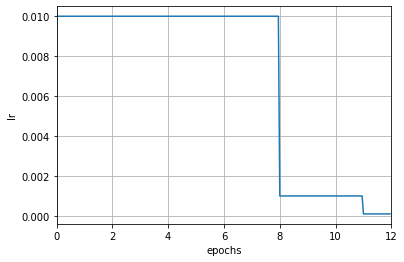If using the runner with the registry and config file, we can specify the scheduler by setting the param_scheduler field in the config. The runner will automatically build a parameter scheduler based on this field:

# build the scheduler with config file
param_scheduler = dict(type='MultiStepLR', by_epoch=True, milestones=[8, 11], gamma=0.1)


Note that the parameter by_epoch is added here, which controls the frequency of learning rate adjustment. When set to True, it means adjusting by epoch. When set to False, it means adjusting by iteration. The default value is True.

In the above example, it means to adjust according to epochs. At this time, the unit of the parameters is epoch. For example, [8, 11] in milestones means that the learning rate will be multiplied by 0.1 at the end of the 8 and 11 epoch.

When the frequency is modified, the meaning of the count-related settings of the scheduler will be changed accordingly. When by_epoch=True, the numbers in milestones indicate at which epoch the learning rate decay is performed, and when by_epoch=False it indicates at which iteration the learning rate decay is performed.

Here is an example of adjusting by iterations: At the end of the 600th and 800th iterations, the learning rate will be multiplied by 0.1 times.

param_scheduler = dict(type='MultiStepLR', by_epoch=False, milestones=[600, 800], gamma=0.1)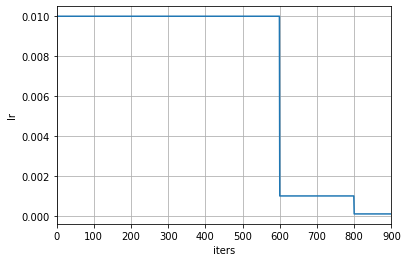If users want to use the iteration-based frequency while filling the scheduler config settings by epoch, MMEngine’s scheduler also provides an automatic conversion method. Users can call the build_iter_from_epoch method and provide the number of iterations for each training epoch to construct a scheduler object updated by iterations:

epoch_length = len(train_dataloader)
param_scheduler = MultiStepLR.build_iter_from_epoch(optimizer, milestones=[8, 11], gamma=0.1, epoch_length=epoch_length)


If using config to build a scheduler, just add convert_to_iter_based=True to the field. The runner will automatically call build_iter_from_epoch to convert the epoch-based config to an iteration-based scheduler object:

param_scheduler = dict(type='MultiStepLR', by_epoch=True, milestones=[8, 11], gamma=0.1, convert_to_iter_based=True)


Below is a Cosine Annealing learning rate scheduler that is updated by epoch, where the learning rate is only modified after each epoch:

param_scheduler = dict(type='CosineAnnealingLR', by_epoch=True, T_max=12)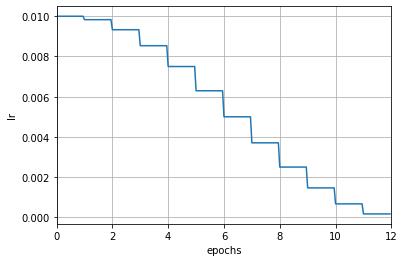After automatically conversion, the learning rate is updated by iteration. As you can see from the graph below, the learning rate changes more smoothly.

param_scheduler = dict(type='CosineAnnealingLR', by_epoch=True, T_max=12, convert_to_iter_based=True)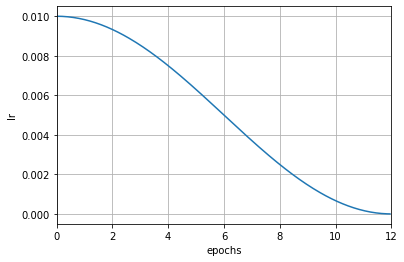### Combine multiple LRSchedulers (e.g. learning rate warm-up)¶

In the training process of some algorithms, the learning rate is not adjusted according to a certain scheduling strategy from beginning to end. The most common example is learning rate warm-up.

For example, in the first few iterations, a linear strategy is used to increase the learning rate from a small value to normal, and then another strategy is applied.

MMEngine supports combining multiple schedulers together. Just modify the param_scheduler field in the config file to a list of scheduler config, and the ParamSchedulerHook can automatically process the scheduler list. The following example implements learning rate warm-up.

param_scheduler = [
# Linear learning rate warm-up scheduler
dict(type='LinearLR',
start_factor=0.001,
by_epoch=False,  # Updated by iterations
begin=0,
end=50),  # Warm up for the first 50 iterations
# The main LRScheduler
dict(type='MultiStepLR',
by_epoch=True,  # Updated by epochs
milestones=[8, 11],
gamma=0.1)
]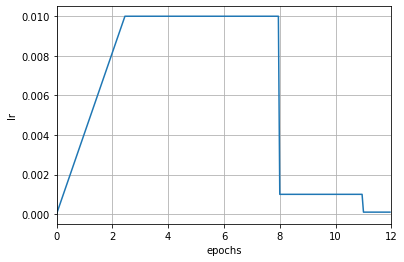Note that the begin and end parameters are added here. These two parameters specify the valid interval of the scheduler. The valid interval usually only needs to be set when multiple schedulers are combined, and can be ignored when using a single scheduler. When the begin and end parameters are specified, it means that the scheduler only takes effect in the [begin, end) interval, and the unit is determined by the by_epoch parameter.

In the above example, the by_epoch of LinearLR in the warm-up phase is False, which means that the scheduler only takes effect in the first 50 iterations. After more than 50 iterations, the scheduler will no longer take effect, and the second scheduler, which is MultiStepLR, will control the learning rate. When combining different schedulers, the by_epoch parameter does not have to be the same for each scheduler.

Here is another example:

param_scheduler = [
# Use a linear warm-up at [0, 100) iterations
dict(type='LinearLR',
start_factor=0.001,
by_epoch=False,
begin=0,
end=100),
# Use a cosine learning rate at [100, 900) iterations
dict(type='CosineAnnealingLR',
T_max=800,
by_epoch=False,
begin=100,
end=900)
]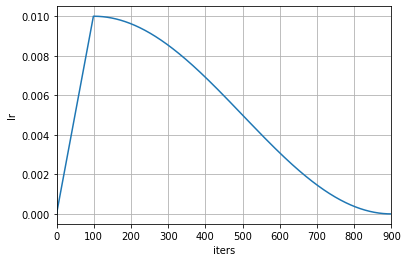The above example uses a linear learning rate warm-up for the first 100 iterations, and then uses a cosine annealing learning rate scheduler with a period of 800 from the 100th to the 900th iteration.

Users can combine any number of schedulers. If the valid intervals of two schedulers are not connected to each other which leads to an interval that is not covered, the learning rate of this interval remains unchanged. If the valid intervals of the two schedulers overlap, the adjustment of the learning rate will be triggered in the order of the scheduler config (similar with ChainedScheduler).

We recommend using different learning rate scheduling strategies in different stages of training to avoid overlapping of the valid intervals. Be careful If you really need to stack two schedulers overlapped. We recommend using learning rate visualization tool to visualize the learning rate after stacking, to avoid the adjustment not as expected.

## How to adjust other hyperparameters¶

### Momentum¶

Like learning rate, momentum is a schedulable hyperparameter in the optimizer’s parameter group. The momentum scheduler is used in exactly the same way as the learning rate scheduler. Just add the momentum scheduler config to the list in the param_scheduler field.

Example:

param_scheduler = [
# the lr scheduler
dict(type='LinearLR', ...),
# the momentum scheduler
dict(type='LinearMomentum',
start_factor=0.001,
by_epoch=False,
begin=0,
end=1000)
]


### Generic parameter scheduler¶

MMEngine also provides a set of generic parameter schedulers for scheduling other hyperparameters in the param_groups of the optimizer. Change LR in the class name of the learning rate scheduler to Param, such as LinearParamScheduler. Users can schedule the specific hyperparameters by setting the param_name variable of the scheduler.

Here is an example:

param_scheduler = [
dict(type='LinearParamScheduler',
param_name='lr',  # adjust the 'lr' in optimizer.param_groups
start_factor=0.001,
by_epoch=False,
begin=0,
end=1000)
]


By setting the param_name to 'lr', this parameter scheduler is equivalent to LinearLRScheduler.

In addition to learning rate and momentum, users can also schedule other parameters in optimizer.param_groups. The schedulable parameters depend on the optimizer used. For example, when using the SGD optimizer with weight_decay, the weight_decay can be adjusted as follows:

param_scheduler = [
dict(type='LinearParamScheduler',
param_name='weight_decay',  # adjust 'weight_decay' in optimizer.param_groups
start_factor=0.001,
by_epoch=False,
begin=0,
end=1000)
]


© Copyright 2022, mmengine contributors. Revision 85c0976b.

Built with Sphinx using a theme provided by Read the Docs.
Versions
latest
stable
v0.10.1
v0.10.0
v0.9.1
v0.9.0
v0.8.5
v0.8.4
v0.8.3
v0.8.2
v0.8.1
v0.8.0
v0.7.4
v0.7.3
v0.7.2
v0.7.1
v0.7.0
v0.6.0
v0.5.0
v0.4.0
v0.3.0
v0.2.0